# NameError: name 'time' or 'datetime' is not defined in PythonLast updated: Feb 2, 2023
10 min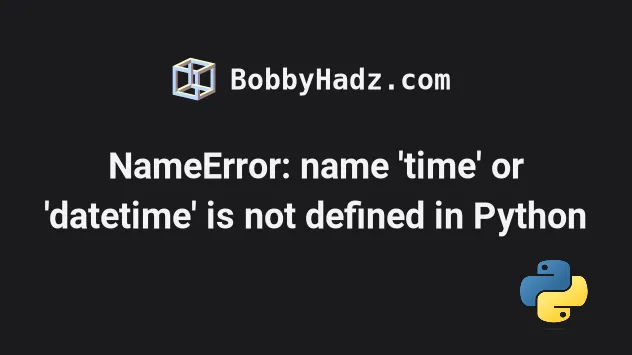Make sure to click on the correct subheading depending on your error message.

## #NameError: name 'time' is not defined in Python

The Python "NameError: name 'time' is not defined" occurs when we use the `time` module without importing it first.

To solve the error, import the `time` module before using it - `import time`.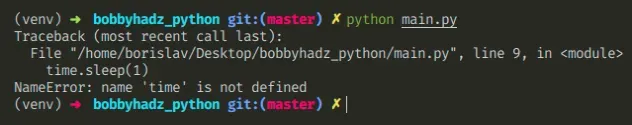Here is an example of how the error occurs.

main.py
```Copied!```# ⛔️ NameError: name 'time' is not defined
time.sleep(1)

print(
time.strftime(
"%a, %d %b %Y %H:%M:%S +0000", time.gmtime()
)
)
``````

## #Import the `time` module before using it

To solve the error, we have to import the time module.

main.py
```Copied!```# 👇️ first import time module
import time

time.sleep(1)

print(
time.strftime(
"%a, %d %b %Y %H:%M:%S +0000", time.gmtime()
)
)
``````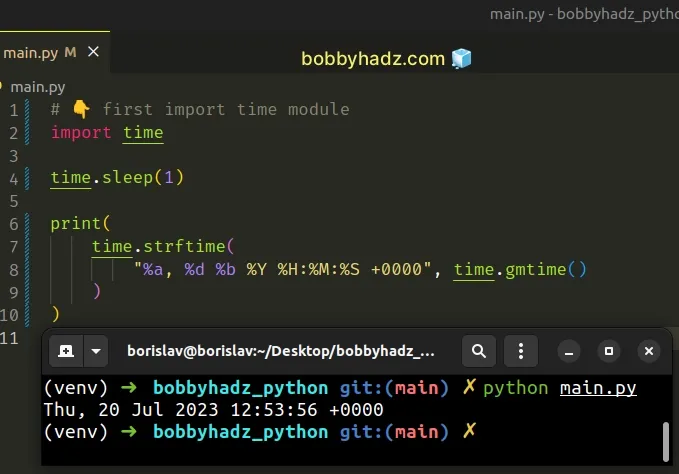Even though the `time` module is in the Python standard library, we still have to import it before using it.

Make sure you haven't used a capital letter `t` when importing `time` because module names are case-sensitive.

## #Make sure to not import the `time` module in a nested scope

Also, make sure you haven't imported `time` in a nested scope, e.g. a function.

main.py
```Copied!```def get_time():
import time

time.sleep(1)

print(
time.strftime(
"%a, %d %b %Y %H:%M:%S +0000", time.gmtime()
)
)

# ⛔️ NameError: name 'time' is not defined
time.sleep(1)
``````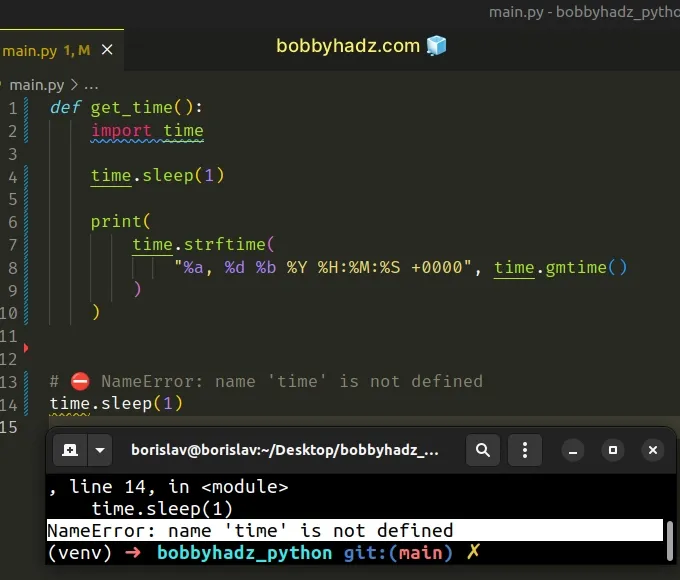We imported the `time` module in a function, so we aren't able to use it outside of the function.

Import the module at the top level to be able to use it throughout your code.

main.py
```Copied!```# ✅ moved import to top level
import time

def get_time():

time.sleep(1)

print(
time.strftime(
"%a, %d %b %Y %H:%M:%S +0000", time.gmtime()
)
)

time.sleep(1)
``````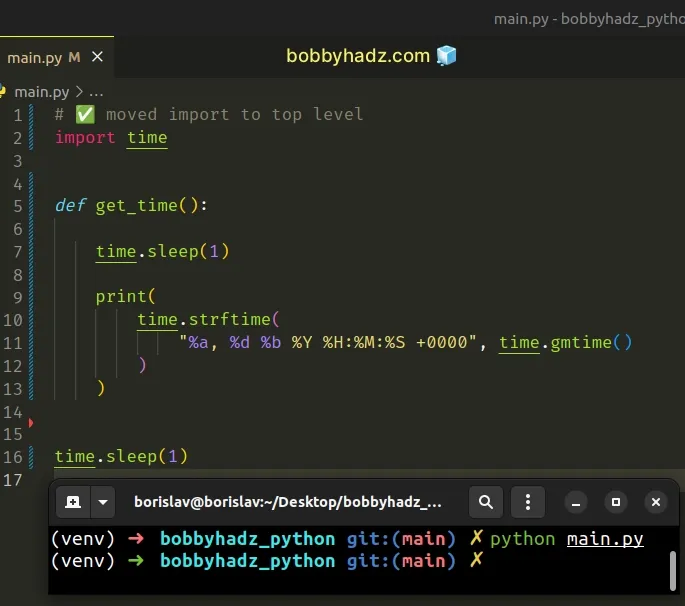The import statement for the `time` module has to come at the top of the file before any code that makes use of it.

## #Make sure to not import the `time` module in a try/except statement

You also should be importing the `time` module in a try/except statement.

main.py
```Copied!```try:
import time

time.sleep(1)

print(
time.strftime(
"%a, %d %b %Y %H:%M:%S +0000", time.gmtime()
)
)
except ImportError:
time.sleep(1)

time.sleep(1)
``````

The code sample works, however, if the code in the `try` statement raises an error, the `time` module won't get imported successfully.

This would cause the error because we are trying to access properties on the `time` module in the outer scope and the `except` block.

Instead, move the import statement to the top of the file.

main.py
```Copied!```# ✅ moved import statement to the top of the file
import time

try:

time.sleep(1)

print(
time.strftime(
"%a, %d %b %Y %H:%M:%S +0000", time.gmtime()
)
)
except ImportError:
time.sleep(1)

time.sleep(1)
``````

## #Only importing the functions you need from the `time` module

Alternatively, you can make your code a little more concise by only importing the functions that you use in your code.

main.py
```Copied!```from time import sleep, strftime, gmtime

sleep(1)

print(
strftime(
"%a, %d %b %Y %H:%M:%S +0000", gmtime()
)
)
``````

The example shows how to import the `sleep()` and `strftime()` and `gmtime` functions from the `time` module.

Instead of accessing the members on the module, e.g. `time.sleep()`, we now access them directly.

For example, when we use an import such as `import time`, it is much harder to see which functions from the `time` module are used in the file.

Conversely, when we import specific functions, it is much easier to see which functions from the `time` module are used.

The `time` module provides many time-related functions.

You can view all of the functions and constants the module provides by visiting the official docs.

## #NameError: name 'sleep' is not defined in Python

If you get an error message such as "NameError: name 'sleep' is not defined", then you have forgotten to import the `sleep` function from the `time` module.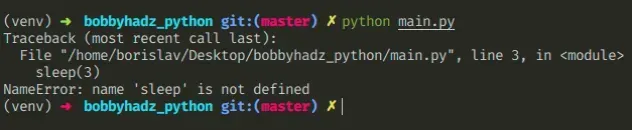Here is an example of how the error occurs.

main.py
```Copied!```# ⛔️ NameError: name 'sleep' is not defined
sleep(3)

print('After 3 seconds')
``````

To solve the error, we have to import the `sleep` function from the `time` module.

main.py
```Copied!```# 👇️ import sleep function
from time import sleep

sleep(3) # 👈️ time in seconds

print('After 3 seconds')
``````

Alternatively, you can import the `time` module and access the `sleep` function as `time.sleep(N)`.

main.py
```Copied!```import time

time.sleep(3)

print('After 3 seconds')
``````

The sleep function takes a number of seconds as a parameter and suspends execution for the given number of seconds.

If you need to call a function or run some logic every N seconds, use a `while` loop.

main.py
```Copied!```import time

while True:
print('Runs every 3 seconds')
time.sleep(3)
``````

The code in the body of the `while` loop is run every `3` seconds.

You can also pass a floating point number as an argument to `sleep` if you need to suspend execution for `X.Y` seconds.

main.py
```Copied!```import time

time.sleep(2.5)

print('After 2.5 seconds')
``````

Note that the `sleep()` function suspends execution at least for the provided number of seconds, except in the case when an exception is raised.

## #NameError: name 'datetime' is not defined in Python

The Python "NameError: name 'datetime' is not defined" occurs when we use the `datetime` module without importing it first.

To solve the error, import the `datetime` module before using it - `import datetime`.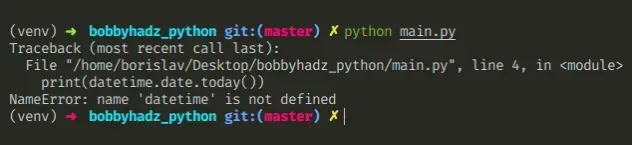Here is an example of how the error occurs.

main.py
```Copied!```# ⛔️ NameError: name 'datetime' is not defined
print(datetime.date.today())

d = datetime.date(2022, 9, 24)
print(d)

t = datetime.time(9, 30)
print(t)
``````

## #Import the `datetime` module before using it

To solve the error, we have to import the datetime module before using it.

main.py
```Copied!```import datetime

print(datetime.date.today())

d = datetime.date(2022, 9, 24)
print(d)  # 👉️ "2022-09-24"

t = datetime.time(9, 30)
print(t)  # 👉️ 09:30:00
``````

Even though the `datetime` module is in the Python standard library, we still have to import it before using it.

Make sure you haven't used a capital letter `d` when importing `datetime` because module names are case-sensitive.

## #Make sure to not import the `datetime` module in a nested scope

Also, make sure you haven't imported `datetime` in a nested scope, e.g. a function.

main.py
```Copied!```def get_datetime():
import datetime

print(datetime.date(2023, 9, 24))

# ⛔️ NameError: name 'datetime' is not defined
print(datetime.date(2023, 9, 24))
``````

We imported the `datetime` module in a function, so we aren't able to use it outside of the function.

Import the module at the top level to be able to use it throughout your code.

main.py
```Copied!```# ✅ moved the import to the top level of the file
import datetime

def get_datetime():

print(datetime.date(2023, 9, 24))

print(datetime.date(2023, 9, 24))
``````

The import statement for the `datetime` module has to come at the top of the file before any code that makes use of it.

## #Don't import the `datetime` module in a `try/except` statement

You also should be importing the `datetime` module in a `try/except` statement.

main.py
```Copied!```try:
# 👉️ code here could raise an error

import datetime

print(datetime.date(2023, 9, 24))
except ImportError:
print(datetime.date(2023, 9, 24))

print(datetime.date(2023, 9, 24))
``````

The code sample works, however, if the code in the `try` statement raises an error, the `datetime` module won't get imported successfully.

This would cause the error because we are trying to access properties on the `datetime` module in the outer scope and the `except` block.

Instead, move the import statement to the top of the file.

main.py
```Copied!```# ✅ moved import statement to the top of the file
import datetime

try:
print(datetime.date(2023, 9, 24))
except ImportError:
print(datetime.date(2023, 9, 24))

print(datetime.date(2023, 9, 24))
``````

## #Importing functions directly from the `datetime` module

Alternatively, you can make your code a little more concise by only importing the classes that you use in your code.

main.py
```Copied!```from datetime import date, time

print(date.today())

d = date(2022, 9, 24)
print(d)  # "2022-09-24"

t = time(9, 30)
print(t)  # 👉️ 09:30:00
``````

The example shows how to import the `date()` and `time()` classes from the `datetime` module.

Instead of accessing the members on the module, e.g. `datetime.date()`, we now access them directly.

For example, when we use an import such as `import datetime`, it is much harder to see which classes from the `datetime` module are being used in the file.

Conversely, when we import specific functions or classes, it is much easier to see which classes from the `datetime` module are being used.

The `datetime` module provides classes for manipulating dates and times.

You can view all of the classes and constants the `datetime` module provides by visiting the official docs.

## #NameError: name 'timedelta' is not defined in Python

The Python "NameError: name 'timedelta' is not defined" occurs when we use the `timedelta` class without importing it first.

To solve the error, import the `timedelta` class before using it - `from datetime import timedelta`.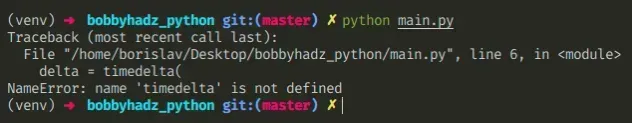Here is an example of how the error occurs.

main.py
```Copied!```# ⛔️ NameError: name 'timedelta' is not defined
delta = timedelta(
days=30,
seconds=45,
microseconds=10,
milliseconds=29000,
minutes=3,
hours=7,
weeks=2,
)

print(delta)

print(delta.max)
print(delta.min)
``````

To solve the error, import the timedelta class from the datetime module.

main.py
```Copied!```# ✅ import timedelta class first
from datetime import timedelta

delta = timedelta(
days=30,
seconds=45,
microseconds=10,
milliseconds=29000,
minutes=3,
hours=7,
weeks=2,
)

print(delta)

print(delta.max)
print(delta.min)
``````

Even though the `datetime` module is in the Python standard library, we still have to import it before using it.

Make sure you haven't misspelled `timedelta` in the import statement as the names of classes are case-sensitive.

Also, make sure you haven't imported `timedelta` in a nested scope, e.g. a function.

Import the function at the top level of the file to be able to use it throughout your code.

A `timedelta` object represents a duration - the difference between two dates or times.

All of the arguments we passed to the class in the example above are optional and default to `0`.

You can read more about `timedelta` objects by visiting the official docs.

## #NameError: name 're' is not defined in Python

The Python "NameError: name 're' is not defined" occurs when we use the `re` module without importing it first.

To solve the error, import the `re` module before using it - `import re`.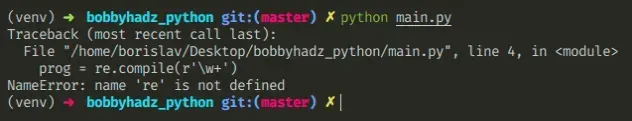Here is an example of how the error occurs.

main.py
```Copied!```# ⛔️ NameError: name 're' is not defined
prog = re.compile(r'\w+')

result = prog.match('hello world 123')

print(result)
``````

## #Import the re module before using it

To solve the error, we have to import the re module.

main.py
```Copied!```# ✅ import the re module first
import re

prog = re.compile(r'\w+')

result = prog.match('hello world 123')

print(result)
``````

Even though the `re` module is in the Python standard library, we still have to import it before using it.

Make sure you haven't used a capital letter `r` when importing `re` because module names are case-sensitive.

## #Make sure to not import the `re` module in a nested scope

Make sure you haven't imported `re` in a nested scope, e.g. a function.

main.py
```Copied!```def example():
import re

prog = re.compile(r'\w+')

result = prog.match('hello world 123')

print(result)

# ⛔️ NameError: name 're' is not defined
prog = re.compile(r'\w+')
``````

We imported the `re` module in a function, so we aren't able to use it outside of the function.

Import the module at the top level to be able to use it throughout your code.

main.py
```Copied!```# ✅ import at the top level of the module
import re

def example():

prog = re.compile(r'\w+')

result = prog.match('hello world 123')

print(result)

# ⛔️ NameError: name 're' is not defined
prog = re.compile(r'\w+')
``````

The import statement for the `re` module has to come at the top of the file before any code that makes use of it.

## #Only importing the functions you need from the `re` module

An alternative to importing the entire `re` module is to import only the functions and constants that your code uses.

main.py
```Copied!```# 👇️ import only compile function from re module
from re import compile

prog = compile(r'\w+')

result = prog.match('hello world 123')

print(result)
``````

The example shows how to only import the `compile` function from the `re` module.

Instead of accessing the members on the module, e.g. `re.compile`, we now access them directly.

For example, when we use an import such as `import re`, it is much harder to see which functions from the `re` module are being used in the file.

Conversely, when we import specific functions, it is much easier to see which functions from the `re` module are being used.

The `re` module provides regular expression matching operations.

You can view all of the functions the `re` module provides by visiting the official docs.

## #NameError: name 'csv' is not defined in Python

The Python "NameError: name 'csv' is not defined" occurs when we use the `csv` module without importing it first.

To solve the error, import the `csv` module before using it - `import csv`.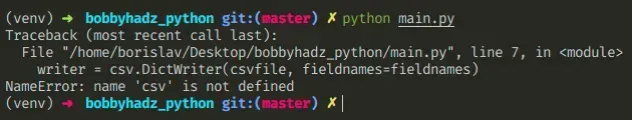Here is an example of how the error occurs.

main.py
```Copied!```with open('employees.csv', 'w', newline='', encoding='utf-8') as csvfile:
fieldnames = ['first_name', 'last_name']
# ⛔️ NameError: name 'csv' is not defined
writer = csv.DictWriter(csvfile, fieldnames=fieldnames)

writer.writerow({'first_name': 'Alice', 'last_name': 'Smith'})
writer.writerow({'first_name': 'Bob', 'last_name': 'Smith'})
writer.writerow({'first_name': 'Carl', 'last_name': 'Smith'})
``````

To solve the error, we have to import the csv module.

main.py
```Copied!```# ✅ import csv module first
import csv

with open('employees.csv', 'w', newline='', encoding='utf-8') as csvfile:
fieldnames = ['first_name', 'last_name']
writer = csv.DictWriter(csvfile, fieldnames=fieldnames)

writer.writerow({'first_name': 'Alice', 'last_name': 'Smith'})
writer.writerow({'first_name': 'Bob', 'last_name': 'Smith'})
writer.writerow({'first_name': 'Carl', 'last_name': 'Smith'})
``````

Even though the `csv` module is in the Python standard library, we still have to import it before using it.

Make sure you haven't used a capital letter `c` when importing `csv` because module names are case-sensitive.

Also, make sure you haven't imported `csv` in a nested scope, e.g. a function. Import the module at the top level to be able to use it throughout your code.

An alternative to importing the entire `csv` module is to import only the functions and classes that your code uses.

main.py
```Copied!```from csv import DictWriter

with open('employees.csv', 'w', newline='', encoding='utf-8') as csvfile:
fieldnames = ['first_name', 'last_name']
writer = DictWriter(csvfile, fieldnames=fieldnames)

writer.writerow({'first_name': 'Alice', 'last_name': 'Smith'})
writer.writerow({'first_name': 'Bob', 'last_name': 'Smith'})
writer.writerow({'first_name': 'Carl', 'last_name': 'Smith'})
``````

The example shows how to import the `DictWriter` class from the `csv` module.

Instead of accessing the members on the module, e.g. `csv.DictWriter`, we now access them directly.

For example, when we use an import such as `import csv`, it is much harder to see which classes or functions from the `csv` module are being used in the file.

Conversely, when we import specific classes, it is much easier to see which classes and methods from the `csv` module are being used.

The `csv` module implements classes to read and write tabular data in CSV format.

You can view all of the classes and methods the `csv` module provides by visiting the official docs.

I wrote a book in which I share everything I know about how to become a better, more efficient programmer.You can use the search field on my Home Page to filter through all of my articles.